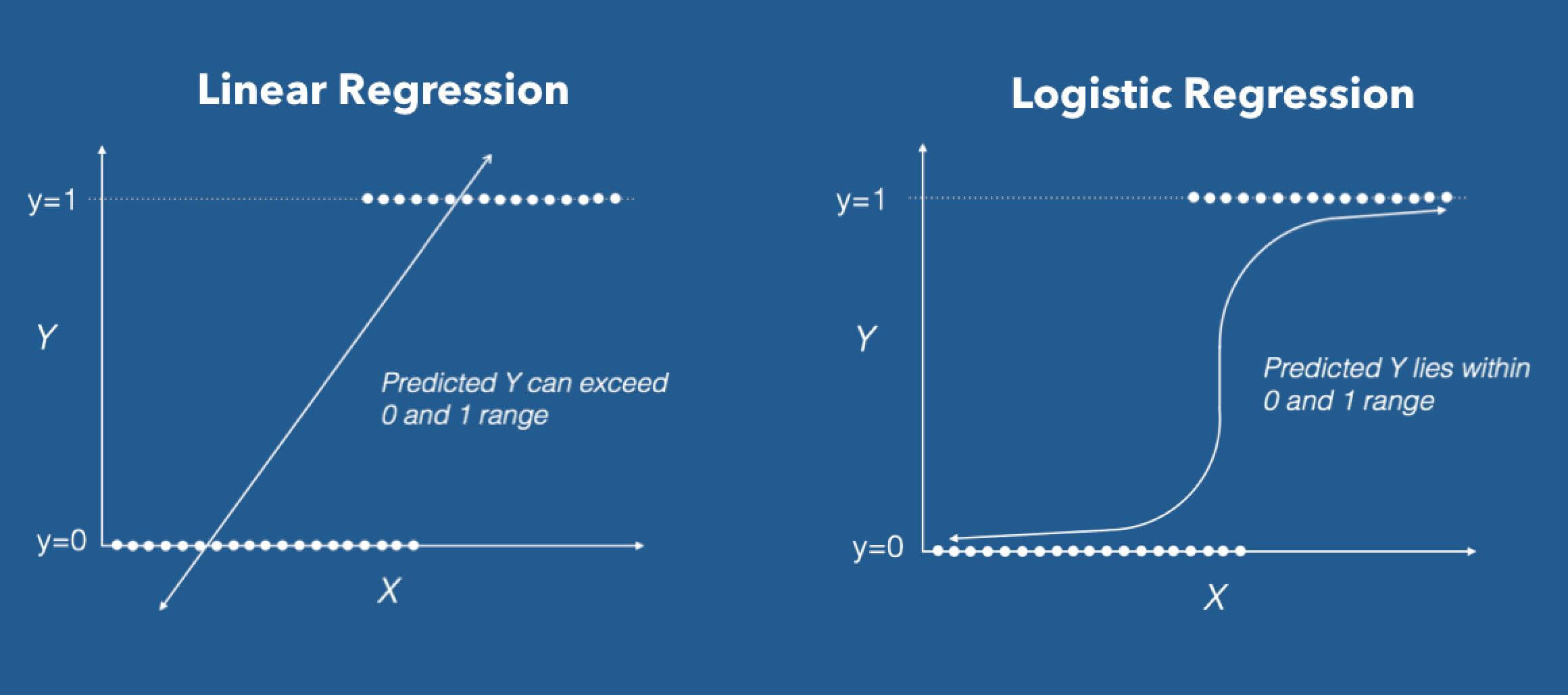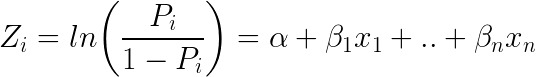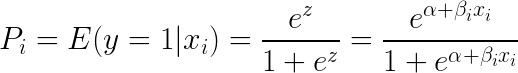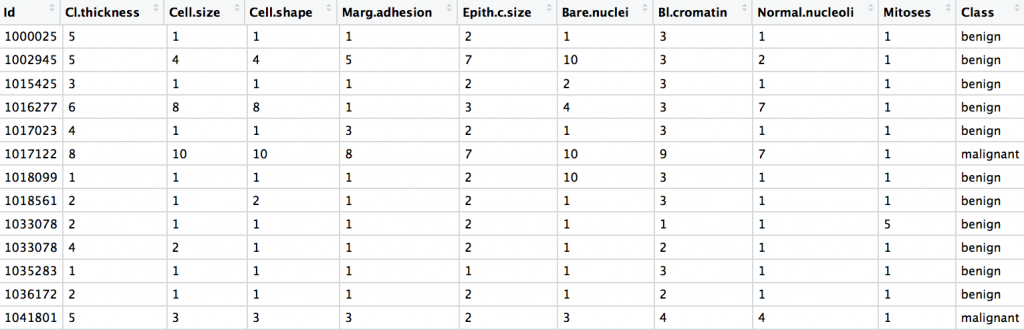# Logistic Regression – A complete Tutorial

Logistic regression is a predictive modelling algorithm that is used when the Y variable is binary categorical. That is, it can take only two values like 1 or 0. The goal is to determine a mathematical equation that can be used to predict the probability of event 1. Once the equation is established, it can be used to predict the Y when only the X’s are known.## Contents

1. Introduction to Logistic Regression
2. Some real world examples of binary classification problems
3. Why not linear regression?
4. The Logistic Equation
5. How to build logistic regression model in R?
6. How to deal with Class Imbalance?
7. How to handle Class Imbalance with Upsample and Downsample?
8. Building the Logistic Regression Model
9. How to Predict on Test Dataset
10. Why handling with class imbalance is important?
11. Conclusion

## 1. Introduction to Logistic Regression

Earlier you saw what is linear regression and how to use it to predict continuous Y variables.

In linear regression the Y variable is always a continuous variable. If suppose, the Y variable was categorical, you cannot use linear regression model it.

So what would you do when the Y is a categorical variable with 2 classes?

Logistic regression can be used to model and solve such problems, also called as binary classification problems.

A key point to note here is that Y can have 2 classes only and not more than that. If Y has more than 2 classes, it would become a multi class classification and you can no longer use the vanilla logistic regression for that.

Yet, Logistic regression is a classic predictive modelling technique and still remains a popular choice for modelling binary categorical variables.

Another advantage of logistic regression is that it computes a prediction probability score of an event. More on that when you actually start building the models.

## 2. Some real world examples of binary classification problems

You might wonder what kind of problems you can use logistic regression for.

Here are some examples of binary classification problems:

• Spam Detection : Predicting if an email is Spam or not
• Credit Card Fraud : Predicting if a given credit card transaction is fraud or not
• Health : Predicting if a given mass of tissue is benign or malignant
• Marketing : Predicting if a given user will buy an insurance product or not
• Banking : Predicting if a customer will default on a loan.## 3. Why not linear regression?

When the response variable has only 2 possible values, it is desirable to have a model that predicts the value either as 0 or 1 or as a probability score that ranges between 0 and 1.

Linear regression does not have this capability. Because, If you use linear regression to model a binary response variable, the resulting model may not restrict the predicted Y values within 0 and 1.This is where logistic regression comes into play. In logistic regression, you get a probability score that reflects the probability of the occurence of the event.

An event in this case is each row of the training dataset. It could be something like classifying if a given email is spam, or mass of cell is malignant or a user will buy a product and so on.

## 4. The Logistic Equation

Logistic regression achieves this by taking the log odds of the event ln(P/1−P), where, P is the probability of event. So P always lies between 0 and 1.Taking exponent on both sides of the equation gives:You can implement this equation using the `glm()` function by setting the `family`argument to `"binomial"`.

````# Template code`
`# Step 1: Build Logit Model on Training Dataset`
logitMod <- glm(Y ~ X1 + X2, family="binomial", data = trainingData)
`# Step 2: Predict Y on Test Dataset`
predictedY <- predict(logitMod, testData, type="response")```

Also, an important caveat is to make sure you set the `type="response"` when using the `predict` function on a logistic regression model. Else, it will predict the log odds of P, that is the Z value, instead of the probability itself.

## 5. How to build logistic regression model in R?

Now let’s see how to implement logistic regression using the `BreastCancer` dataset in `mlbench` package. You will have to install the `mlbench` package for this.

The goal here is to model and predict if a given specimen (row in dataset) is `benign` or `malignant`, based on 9 other cell features. So, let’s load the data and keep only the complete cases.

````# install.packages("mlbench")`
data(BreastCancer, package="mlbench")
bc <- BreastCancer[complete.cases(BreastCancer), ] `# create copy````The dataset has 699 observations and 11 columns. The `Class` column is the response (dependent) variable and it tells if a given tissue is malignant or benign.

Let’s check the structure of this dataset.

```str(bc)
```#> 'data.frame':	683 obs. of  11 variables:
#> \$ Id             : chr  "1000025" "1002945" "1015425" "1016277" ...
#> \$ Cl.thickness   : Ord.factor w/ 10 levels "1"<"2"<"3"<"4"<..: 5 5 3 6 4 8 1 2 2 4 ...
#> \$ Cell.size      : Ord.factor w/ 10 levels "1"<"2"<"3"<"4"<..: 1 4 1 8 1 10 1 1 1 2 ...
#> \$ Cell.shape     : Ord.factor w/ 10 levels "1"<"2"<"3"<"4"<..: 1 4 1 8 1 10 1 2 1 1 ...
#> \$ Marg.adhesion  : Ord.factor w/ 10 levels "1"<"2"<"3"<"4"<..: 1 5 1 1 3 8 1 1 1 1 ...
#> \$ Epith.c.size   : Ord.factor w/ 10 levels "1"<"2"<"3"<"4"<..: 2 7 2 3 2 7 2 2 2 2 ...
#> \$ Bare.nuclei    : Factor w/ 10 levels "1","2","3","4",..: 1 10 2 4 1 10 10 1 1 1 ...
#> \$ Bl.cromatin    : Factor w/ 10 levels "1","2","3","4",..: 3 3 3 3 3 9 3 3 1 2 ...
#> \$ Normal.nucleoli: Factor w/ 10 levels "1","2","3","4",..: 1 2 1 7 1 7 1 1 1 1 ...
#> \$ Mitoses        : Factor w/ 9 levels "1","2","3","4",..: 1 1 1 1 1 1 1 1 5 1 ...
#> \$ Class          : Factor w/ 2 levels "benign","malignant": 1 1 1 1 1 2 1 1 1 1 ...``````

Except `Id`, all the other columns are factors. This is a problem when you model this type of data.

Because, when you build a logistic model with factor variables as features, it converts each level in the factor into a dummy binary variable of 1’s and 0’s.

For example, Cell shape is a factor with 10 levels. When you use glm to model Class as a function of cell shape, the cell shape will be split into 9 different binary categorical variables before building the model.

If you are to build a logistic model without doing any preparatory steps then the following is what you might do. But we are not going to follow this as there are certain things to take care of before building the logit model.

The syntax to build a logit model is very similar to the `lm` function you saw in linear regression. You only need to set the `family='binomial'` for `glm` to build a logistic regression model.

`glm` stands for generalised linear models and it is capable of building many types of regression models besides linear and logistic regression.

Lets see how the code to build a logistic model might look like. I will be coming to this step again later as there are some preprocessing steps to be done before building the model.

```glm(Class ~ Cell.shape, family="binomial", data = bc)
```#> Call:  glm(formula = Class ~ Cell.shape, family = "binomial", data = bc)

#> Coefficients:
#>  (Intercept)  Cell.shape.L  Cell.shape.Q  Cell.shape.C
#>        4.189        20.911         6.848         5.763
#> Cell.shape^4  Cell.shape^5  Cell.shape^6  Cell.shape^7
#>       -1.267        -4.439        -5.183        -3.013
#> Cell.shape^8  Cell.shape^9
#>       -1.289        -0.860

#> Degrees of Freedom: 682 Total (i.e. Null);  673 Residual
#> Null Deviance:	    884.4
#> Residual Deviance: 243.6 	AIC: 263.6``````

In above model, `Class` is modeled as a function of `Cell.shape` alone.

But note from the output, the `Cell.Shape` got split into 9 different variables. This is because, since `Cell.Shape` is stored as a factor variable, `glm` creates 1 binary variable (a.k.a dummy variable) for each of the 10 categorical level of `Cell.Shape`.

Clearly, from the meaning of `Cell.Shape` there seems to be some sort of ordering within the categorical levels of `Cell.Shape`. That is, a cell shape value of 2 is greater than cell shape 1 and so on.

This is the case with other variables in the dataset a well. So, its preferable to convert them into numeric variables and remove the id column.

Had it been a pure categorical variable with no internal ordering, like, say the sex of the patient, you may leave that variable as a factor itself.

````# remove id column`
bc <- bc[,-1]

`# convert factors to numeric`
for(i in 1:9) { bc[, i] <- as.numeric(as.character(bc[, i])) }```

Another important point to note. When converting a factor to a numeric variable, you should always convert it to character and then to numeric, else, the values can get screwed up.

Now all the columns are numeric.

Also I’d like to encode the response variable into a factor variable of 1’s and 0’s. Though, this is only an optional step.

So whenever the `Class` is `malignant`, it will be 1 else it will be 0. Then, I am converting it into a factor.

```bc\$Class <- ifelse(bc\$Class == "malignant", 1, 0)
bc\$Class <- factor(bc\$Class, levels = c(0, 1))```

The response variable `Class` is now a factor variable and all other columns are numeric.

Alright, the classes of all the columns are set. Let’s proceed to the next step.

## 6. How to deal with Class Imbalance?

Before building the logistic regressor, you need to randomly split the data into training and test samples.

Since the response variable is a binary categorical variable, you need to make sure the training data has approximately equal proportion of classes.```table(bc\$Class)
```#>   benign malignant
#>      444       239``````

The classes ‘benign’ and ‘malignant’ are split approximately in 1:2 ratio.

Clearly there is a class imbalance. So, before building the logit model, you need to build the samples such that both the 1’s and 0’s are in approximately equal proportions.

This concern is normally handled with a couple of techniques called:

So, what is Down Sampling and Up Sampling?

## 7. How to handle Class Imbalance with Upsampling and Downsampling

In Down sampling, the majority class is randomly down sampled to be of the same size as the smaller class. That means, when creating the training dataset, the rows with the benign Class will be picked fewer times during the random sampling.

Similarly, in UpSampling, rows from the minority class, that is, `malignant` is repeatedly sampled over and over till it reaches the same size as the majority class (`benign`).

But in case of Hybrid sampling, artificial data points are generated and are systematically added around the minority class. This can be implemented using the `SMOTE` and `ROSE`packages.

However for this example, I will show how to do up and down sampling.

So let me create the Training and Test Data using caret Package.

```library(caret)
'%ni%' <- Negate('%in%')  `# define 'not in' func`
options(scipen=999) `# prevents printing scientific notations.`

`# Prep Training and Test data.`
set.seed(100)
trainDataIndex <- createDataPartition(bc\$Class, p=0.7, list = F) `# 70% training data`
trainData <- bc[trainDataIndex, ]
testData <- bc[-trainDataIndex, ]```

In the above snippet, I have loaded the caret package and used the `createDataPartition` function to generate the row numbers for the training dataset. By setting `p=.70`I have chosen 70% of the rows to go inside `trainData` and the remaining 30% to go to `testData`.

`table(trainData\$Class)`

There is approximately 2 times more benign samples. So lets downsample it using the `downSample` function from `caret` package.

To do this you just need to provide the X and Y variables as arguments.

````# Down Sample`
set.seed(100)
down_train <- downSample(x = trainData[, colnames(trainData) %ni% "Class"], y = trainData\$Class)

table(down_train\$Class)
```#>   benign malignant
#>      168       168``````

Benign and malignant are now in the same ratio.

The `%ni%` is the negation of the `%in%` function and I have used it here to select all the columns except the `Class` column.

The `downSample` function requires the ‘y’ as a factor variable, that is reason why I had converted the class to a factor in the original data.

Great! Now let me do the upsampling using the `upSample` function. It follows a similar syntax as `downSample`.

````# Up Sample.`
set.seed(100)
up_train <- upSample(x = trainData[, colnames(trainData) %ni% "Class"], y = trainData\$Class)

table(up_train\$Class)
```#>   benign malignant
#>      311       311``````

As expected, benign and malignant are now in the same ratio.

I will use the downSampled version of the dataset to build the logit model in the next step.

## 8. Building the Logistic Regression Model

````# Build Logistic Model` logitmod <- glm(Class ~ Cl.thickness + Cell.size + Cell.shape, family = "binomial", data=down_train) summary(logitmod) ```#> Call:
#> glm(formula = Class ~ Cl.thickness + Cell.size + Cell.shape,
#>     family = "binomial", data = down_train)
#>
#> Deviance Residuals:
#>     Min       1Q   Median       3Q      Max
#> -2.1136  -0.0781  -0.0116   0.0000   3.9883

#> Coefficients:
#>                 Estimate Std. Error z value Pr(>|z|)
#> (Intercept)       21.942   3949.431   0.006    0.996
#> Cl.thickness.L    24.279   5428.207   0.004    0.996
#> Cl.thickness.Q    14.068   3609.486   0.004    0.997
#> Cl.thickness.C     5.551   3133.912   0.002    0.999
#> Cl.thickness^4    -2.409   5323.267   0.000    1.000
#> Cl.thickness^5    -4.647   6183.074  -0.001    0.999
#> Cl.thickness^6    -8.684   5221.229  -0.002    0.999
#> Cl.thickness^7    -7.059   3342.140  -0.002    0.998
#> Cl.thickness^8    -2.295   1586.973  -0.001    0.999
#> Cl.thickness^9    -2.356    494.442  -0.005    0.996
#> Cell.size.L       28.330   9300.873   0.003    0.998
#> Cell.size.Q       -9.921   6943.858  -0.001    0.999
#> Cell.size.C       -6.925   6697.755  -0.001    0.999
#> Cell.size^4        6.348  10195.229   0.001    1.000
#> Cell.size^5        5.373  12153.788   0.000    1.000
#> Cell.size^6       -3.636  10824.940   0.000    1.000
#> Cell.size^7        1.531   8825.361   0.000    1.000
#> Cell.size^8        7.101   8508.873   0.001    0.999
#> Cell.size^9       -1.820   8537.029   0.000    1.000
#> Cell.shape.L      10.884   9826.816   0.001    0.999
#> Cell.shape.Q      -4.424   6049.000  -0.001    0.999
#> Cell.shape.C       5.197   6462.608   0.001    0.999
#> Cell.shape^4      12.961  10633.171   0.001    0.999
#> Cell.shape^5       6.114  12095.497   0.001    1.000
#> Cell.shape^6       2.716  11182.902   0.000    1.000
#> Cell.shape^7       3.586   8973.424   0.000    1.000
#> Cell.shape^8      -2.459   6662.174   0.000    1.000
#> Cell.shape^9     -17.783   5811.352  -0.003    0.998

#> (Dispersion parameter for binomial family taken to be 1)

#>     Null deviance: 465.795  on 335  degrees of freedom
#> Residual deviance:  45.952  on 308  degrees of freedom
#> AIC: 101.95

#> Number of Fisher Scoring iterations: 21``````

## 9. How to Predict on Test Dataset

The `logitmod` is now built. You can now use it to predict the response on `testData`.

`pred <- predict(logitmod, newdata = testData, type = "response")`

Now, `pred` contains the probability that the observation is malignant for each observation.

Note that, when you use logistic regression, you need to set `type='response'` in order to compute the prediction probabilities. This argument is not needed in case of linear regression.

The common practice is to take the probability cutoff as 0.5. If the probability of Y is > 0.5, then it can be classified an event (malignant).

So if pred is greater than 0.5, it is malignant else it is benign.

```y_pred_num <- ifelse(pred > 0.5, 1, 0)
y_pred <- factor(y_pred_num, levels=c(0, 1))
y_act <- testData\$Class```

Let’s compute the accuracy, which is nothing but the proportion of `y_pred` that matches with `y_act`.

```mean(y_pred == y_act)  `# 94+%`
`#>  0.9411765````

There you have an accuracy rate of 94%.

## 10. Why handling with class imbalance is important?

Alright I promised I will tell you why you need to take care of class imbalance earlier. To understand that lets assume you have a dataset where 95% of the Y values belong to benign class and 5% belong to malignant class.

Had I just blindly predicted all the data points as benign, I would achieve an accuracy percentage of 95%. Which sounds pretty high. But obviously that is flawed. What matters is how well you predict the malignant classes.

So that requires the benign and malignant classes are balanced AND on top of that I need more refined accuracy measures and model evaluation metrics to improve my prediction model.

## Full Code

``````# Load data
# install.packages('mlbench')```
data(BreastCancer, package="mlbench")
bc <- BreastCancer[complete.cases(BreastCancer), ] ` # keep complete rows`

`# remove id column`
bc <- bc[,-1]

`# convert to numeric`
for(i in 1:9) {
bc[, i] <- as.numeric(as.character(bc[, i]))
}

`# Change Y values to 1's and 0's`
bc\$Class <- ifelse(bc\$Class == "malignant", 1, 0)
bc\$Class <- factor(bc\$Class, levels = c(0, 1)) ```

# Prep Training and Test data.```
library(caret)
'%ni%' <- Negate('%in%') `# define 'not in' func`
options(scipen=999) `# prevents printing scientific notations.`
set.seed(100)
trainDataIndex <- createDataPartition(bc\$Class, p=0.7, list = F)
trainData <- bc[trainDataIndex, ]
testData <- bc[-trainDataIndex, ]

`# Class distribution of train data`
table(trainData\$Class)

`# Down Sample`
set.seed(100)
down_train <- downSample(x = trainData[, colnames(trainData) %ni% "Class"], y = trainData\$Class)
table(down_train\$Class)

`# Up Sample (optional)`
set.seed(100)
up_train <- upSample(x = trainData[, colnames(trainData) %ni% "Class"], y = trainData\$Class)
table(up_train\$Class)

`# Build Logistic Model`
logitmod <- glm(Class ~ Cl.thickness + Cell.size + Cell.shape, family = "binomial", data=down_train)
summary(logitmod)

pred <- predict(logitmod, newdata = testData, type = "response")
pred

`# Recode factors`
y_pred_num <- ifelse(pred > 0.5, 1, 0)
y_pred <- factor(y_pred_num, levels=c(0, 1))
y_act <- testData\$Class

`# Accuracy`
mean(y_pred == y_act) # 94%```

## 11. Conclusion

In this post you saw when and how to use logistic regression to classify binary response variables in R.

You saw this with an example based on the `BreastCancer` dataset where the goal was to determine if a given mass of tissue is malignant or benign.

Building the model and classifying the Y is only half work done. Actually, not even half. Because, the scope of evaluation metrics to judge the efficacy of the model is vast and requires careful judgement to choose the right model. In the next part, I will discuss various evaluation metrics that will help to understand how well the classification model performs from different perspectives.